# Difference between revisions of "Riemannian connection"

An affine connection on a Riemannian spacewith respect to which the metric tensor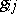of the space is covariantly constant. If the affine connection onis given by a matrix of local connection forms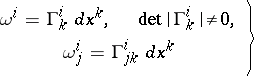(1)

and the metric form onis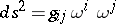, then the latter condition is expressed as(2)

It can be also expressed as follows: Under parallel displacement along any curve in, the scalar product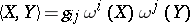of two arbitrary vectors preserves its value, i.e. for vector fields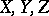onthe following equality holds: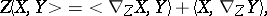where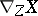is the vector field, called the covariant derivative of the fieldrelative to the field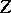, defined by the formula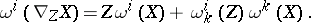If inone goes over to a local field of orthonormal frames, then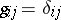(if one restricts to the case of a positive-definite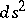) and condition (2) takes the form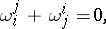i.e. the matrixof forms (1) takes values in the Lie algebra of the group of motions of the Euclidean spaceof dimension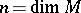. Thus, a Riemannian connection can be interpreted as a connection in the fibre space of orthonormal frames in the Euclidean spaces tangent to. The holonomy group of a Riemannian connection is a subgroup of the group of motions of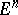; a Riemannian connection for some Riemannian metric onis any affine connection whose holonomy group is the group of motions or some subgroup of it.

If in (1)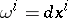(i.e.is considered with respect to the field of natural frames of a local coordinate system), then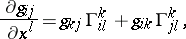and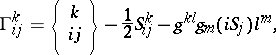where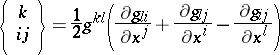is the so-called Christoffel symbol and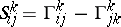is the torsion tensor of the Riemannian connection. There exists one and only one Riemannian connection without torsion (i.e. such that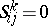); it is determined by the forms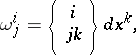and it is called the Levi-Civita connection.

How to Cite This Entry:
Riemannian connection. Encyclopedia of Mathematics. URL: http://encyclopediaofmath.org/index.php?title=Riemannian_connection&oldid=49405
This article was adapted from an original article by Ãœ. Lumiste (originator), which appeared in Encyclopedia of Mathematics - ISBN 1402006098. See original article